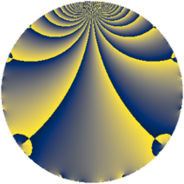Properties

 Label 663.2.aLevel $663$ Weight $2$ Character orbit 663.a Rep. character $\chi_{663}(1,\cdot)$ Character field $\Q$ Dimension $31$ Newform subspaces $9$ Sturm bound $168$ Trace bound $3$

Related objects

Defining parameters

 Level: $$N$$ $$=$$ $$663 = 3 \cdot 13 \cdot 17$$ Weight: $$k$$ $$=$$ $$2$$ Character orbit: $$[\chi]$$ $$=$$ 663.a (trivial) Character field: $$\Q$$ Newform subspaces: $$9$$ Sturm bound: $$168$$ Trace bound: $$3$$ Distinguishing $$T_p$$: $$2$$, $$5$$

Dimensions

The following table gives the dimensions of various subspaces of $$M_{2}(\Gamma_0(663))$$.

Total New Old
Modular forms 88 31 57
Cusp forms 81 31 50
Eisenstein series 7 0 7

The following table gives the dimensions of the cuspidal new subspaces with specified eigenvalues for the Atkin-Lehner operators and the Fricke involution.

$$3$$$$13$$$$17$$FrickeDim.
$$+$$$$+$$$$+$$$$+$$$$3$$
$$+$$$$+$$$$-$$$$-$$$$7$$
$$+$$$$-$$$$+$$$$-$$$$5$$
$$+$$$$-$$$$-$$$$+$$$$1$$
$$-$$$$+$$$$+$$$$-$$$$5$$
$$-$$$$+$$$$-$$$$+$$$$1$$
$$-$$$$-$$$$+$$$$+$$$$3$$
$$-$$$$-$$$$-$$$$-$$$$6$$
Plus space$$+$$$$8$$
Minus space$$-$$$$23$$

Trace form

 $$31 q + 5 q^{2} - q^{3} + 33 q^{4} - 6 q^{5} + q^{6} + 8 q^{7} + 33 q^{8} + 31 q^{9} + O(q^{10})$$ $$31 q + 5 q^{2} - q^{3} + 33 q^{4} - 6 q^{5} + q^{6} + 8 q^{7} + 33 q^{8} + 31 q^{9} + 22 q^{10} + 4 q^{11} + q^{12} - q^{13} + 8 q^{14} + 2 q^{15} + 33 q^{16} - q^{17} + 5 q^{18} - 20 q^{19} - 18 q^{20} - 8 q^{21} - 4 q^{22} - 3 q^{24} + 33 q^{25} - 3 q^{26} - q^{27} + 16 q^{28} + 10 q^{29} - 10 q^{30} + 41 q^{32} + 12 q^{33} + 5 q^{34} + 24 q^{35} + 33 q^{36} + 2 q^{37} - 4 q^{38} + 7 q^{39} + 6 q^{40} - 18 q^{41} - 16 q^{42} + 20 q^{43} + 12 q^{44} - 6 q^{45} + 24 q^{46} + 48 q^{47} - 15 q^{48} + 7 q^{49} + 35 q^{50} - q^{51} + q^{52} + 18 q^{53} + q^{54} - 8 q^{55} - 12 q^{57} - 42 q^{58} - 20 q^{59} - 18 q^{60} - 38 q^{61} - 32 q^{62} + 8 q^{63} + 73 q^{64} + 2 q^{65} + 12 q^{66} + 28 q^{67} - 7 q^{68} - 16 q^{69} - 64 q^{70} - 16 q^{71} + 33 q^{72} + 6 q^{73} - 2 q^{74} - 15 q^{75} - 92 q^{76} - 24 q^{77} + q^{78} - 32 q^{79} - 114 q^{80} + 31 q^{81} - 6 q^{82} - 44 q^{83} - 32 q^{84} + 2 q^{85} + 20 q^{86} - 6 q^{87} - 28 q^{88} - 34 q^{89} + 22 q^{90} + 8 q^{91} - 32 q^{92} - 16 q^{93} - 72 q^{94} + 24 q^{95} - 35 q^{96} + 14 q^{97} - 3 q^{98} + 4 q^{99} + O(q^{100})$$

Decomposition of $$S_{2}^{\mathrm{new}}(\Gamma_0(663))$$ into newform subspaces

Label Dim. $$A$$ Field CM Traces A-L signs $q$-expansion
$a_{2}$ $a_{3}$ $a_{5}$ $a_{7}$ 3 13 17
663.2.a.a $1$ $5.294$ $$\Q$$ None $$-1$$ $$-1$$ $$-2$$ $$0$$ $+$ $-$ $-$ $$q-q^{2}-q^{3}-q^{4}-2q^{5}+q^{6}+3q^{8}+\cdots$$
663.2.a.b $1$ $5.294$ $$\Q$$ None $$-1$$ $$1$$ $$0$$ $$-2$$ $-$ $+$ $-$ $$q-q^{2}+q^{3}-q^{4}-q^{6}-2q^{7}+3q^{8}+\cdots$$
663.2.a.c $1$ $5.294$ $$\Q$$ None $$1$$ $$-1$$ $$-4$$ $$2$$ $+$ $+$ $-$ $$q+q^{2}-q^{3}-q^{4}-4q^{5}-q^{6}+2q^{7}+\cdots$$
663.2.a.d $3$ $5.294$ 3.3.148.1 None $$-3$$ $$3$$ $$-6$$ $$-2$$ $-$ $-$ $+$ $$q+(-1-\beta _{2})q^{2}+q^{3}+(2-\beta _{1}+\beta _{2})q^{4}+\cdots$$
663.2.a.e $3$ $5.294$ 3.3.148.1 None $$-1$$ $$-3$$ $$0$$ $$-4$$ $+$ $+$ $+$ $$q-\beta _{1}q^{2}-q^{3}+(\beta _{1}+\beta _{2})q^{4}+\beta _{1}q^{6}+\cdots$$
663.2.a.f $5$ $5.294$ 5.5.1004368.1 None $$1$$ $$-5$$ $$2$$ $$8$$ $+$ $-$ $+$ $$q+\beta _{1}q^{2}-q^{3}+(1+\beta _{2})q^{4}-\beta _{4}q^{5}+\cdots$$
663.2.a.g $5$ $5.294$ 5.5.153424.1 None $$3$$ $$5$$ $$0$$ $$2$$ $-$ $+$ $+$ $$q+(1+\beta _{3})q^{2}+q^{3}+(2+\beta _{3}-\beta _{4})q^{4}+\cdots$$
663.2.a.h $6$ $5.294$ 6.6.83831632.1 None $$2$$ $$-6$$ $$0$$ $$2$$ $+$ $+$ $-$ $$q+\beta _{1}q^{2}-q^{3}+(1+\beta _{1}+\beta _{2})q^{4}+(-\beta _{2}+\cdots)q^{5}+\cdots$$
663.2.a.i $6$ $5.294$ 6.6.15187408.1 None $$4$$ $$6$$ $$4$$ $$2$$ $-$ $-$ $-$ $$q+(1-\beta _{1})q^{2}+q^{3}+(1-\beta _{1}+\beta _{2})q^{4}+\cdots$$

Decomposition of $$S_{2}^{\mathrm{old}}(\Gamma_0(663))$$ into lower level spaces

$$S_{2}^{\mathrm{old}}(\Gamma_0(663)) \cong$$ $$S_{2}^{\mathrm{new}}(\Gamma_0(17))$$$$^{\oplus 4}$$$$\oplus$$$$S_{2}^{\mathrm{new}}(\Gamma_0(39))$$$$^{\oplus 2}$$$$\oplus$$$$S_{2}^{\mathrm{new}}(\Gamma_0(51))$$$$^{\oplus 2}$$$$\oplus$$$$S_{2}^{\mathrm{new}}(\Gamma_0(221))$$$$^{\oplus 2}$$